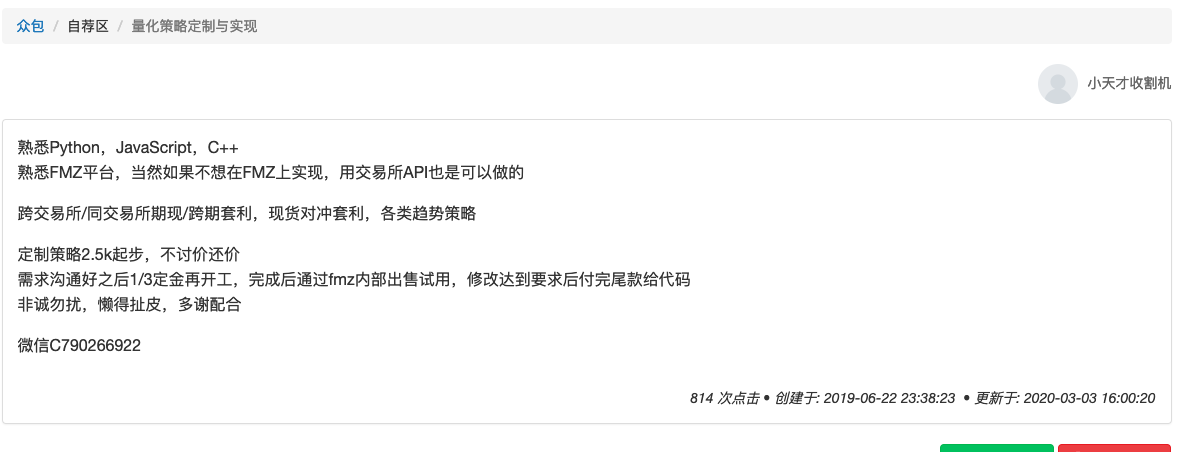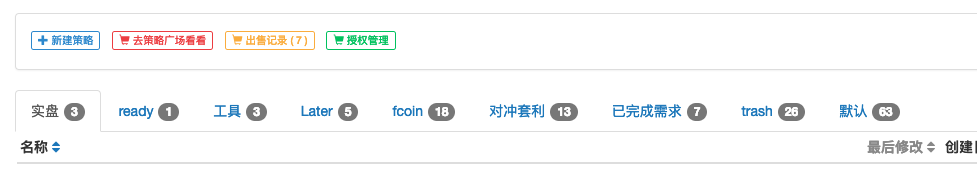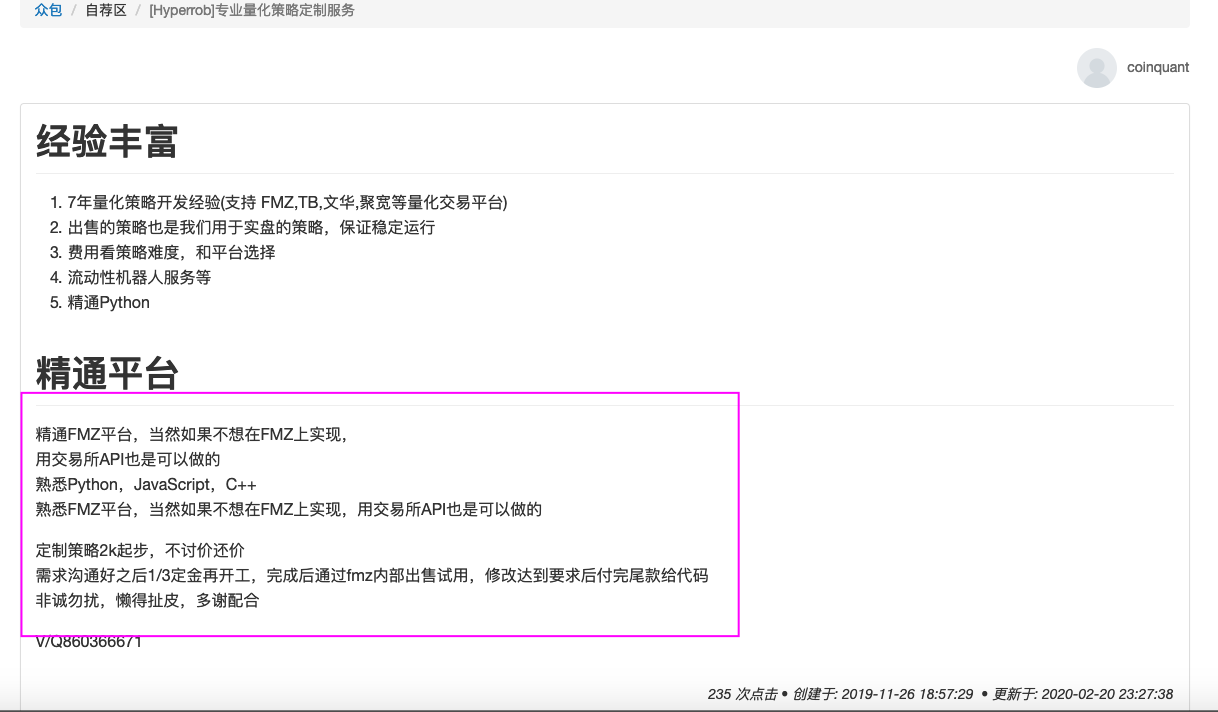# 98年本科生的炒币和量化之路

### 1.入币圈

9月1号，我在一个微信公众号上看到了一篇介绍比特币的视频（好像是王自如的视频），对这个东西感到十分好奇，于是下载了火币，充了100块钱进去，一看比特币价格，3w多人民币，吓到我了，所以就买了一点LTC，当时好像一个120人民币左右，接下来一晚上就盯着账户里那几毛钱一块钱的浮动，以及在加的几个币圈qq群里的看人聊天。盯了一晚上，群友们都说要涨，我也觉得不够刺激，就又充了几千块钱进去，那时候火币还是银行卡充值，过了很久都没到账，我慌了，向群友求助，打客服电话，过了段时间总算是到账了，然后我又全买了LTC。

9月4号，央行一发文，币价跳水，吓得我赶紧割肉离场，然后慢慢地看着币价腰斩，那时候火币等等国内的交易所都不能注册了，国内外交易所差价巨大，肥了一波搬砖的人。

18年1月，轰轰烈烈的牛市开始落幕，我注册了okcoin，开始玩合约，不得不说是真的刺激，20倍的杠杆，翻倍，爆仓，如此往复，盯着k线，夜夜不眠。

### 3.用FMZ### 4.一个套利思路

``````USDollar/BritishPound 0.5
BritishPound/FrenchFranc 10.0
FrenchFranc/USDollar 0.21
``````

``````USDollar/BritishPound 0.5
USDollar/FrenchFranc 4.9
BritishPound/FrenchFranc 10.0
BritishPound/USDollar 1.99
FrenchFranc/BritishPound 0.09
FrenchFranc/USDollar 0.19
``````

``````var coins = {
EMPTY: 0,
};
var price = {};

function get_coins() {
for(var i = 0; i < exchanges.length; ++i) {
var base = _C(exchanges[i].GetCurrency);
var quote = _C(exchanges[i].GetQuoteCurrency);
var len = Object.keys(coins).length;
if(!coins.hasOwnProperty(quote)) {
coins[quote] = len;
len += 1;
}
if(!coins.hasOwnProperty(base)) {
coins[base] = len;
len += 1;
}
}
}

function get_graph() {
var graph = [];
for(var i = 0; i < exchanges.length; ++i) {
var ticker = _C(exchanges[i].GetTicker);
var base = _C(exchanges[i].GetCurrency);
var quote = _C(exchanges[i].GetQuoteCurrency);
graph.push({
from: coins[base],
to: coins[quote],
});
graph.push({
from: coins[quote],
to: coins[base],
weight: 1 / ticker.Sell
});
price[quote + base] = 1 / ticker.Sell;
}
return graph;
}

function bfm(graph, initAmount, feeRate) {
var dist = [];
var pre = [];
var i;
var j;
var fee = 0;
for(i = 0; i < Object.keys(coins).length; ++i) {
dist.push(0);
pre.push(-1);
}
dist = initAmount;
for(i = 0; i < Object.keys(coins).length - 1; ++i) {
for(j = 0; j < graph.length; ++j) {
var from = graph[j].from;
var to = graph[j].to;
var rate = graph[j].weight;
if(dist[from] * rate > dist[to]) {
dist[to] = dist[from] * rate;
pre[to] = from;
}
}
}
if(dist <= initAmount) {
return false;
}
var path = [];
var top = pre;
var coin_keys = Object.keys(coins);
path.push({
from: coin_keys[top],
to: coin_keys,
rate: price[coin_keys[top] + coin_keys]
});
while(top != 1) {
var temp = top;
top = pre[top];
path.push({
from: coin_keys[top],
to: coin_keys[temp],
rate: price[coin_keys[top] + coin_keys[temp]],
});
}
path.reverse();

fee = path.length * feeRate * initAmount;
if(dist - initAmount <= fee || path.length == 0) {
return false;
}
return path;
}

function onTick() {
var graph = get_graph();
var account = _C(exchanges.GetAccount);
var balance = account.Balance;

var path = bfm(graph, balance, 0);
if(!path) {
return;
}
Log(path);

...
}

function main() {
get_coins();

while(1) {
onTick();
}
}
``````